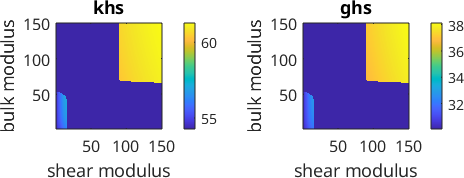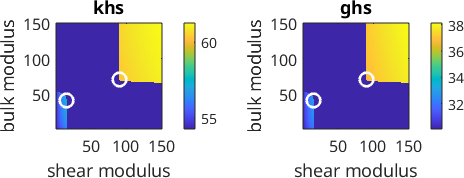Isotropic Theory edit page

While the linear elastic model for anisotropic materials is based on the fourth order elastic stiffness tensor C the linear elastic model for isotropic models is most often developed in terms of the elastic moduli shear, bulk, Youngs modulus and the Poisson ratio.

## The single crystal stiffness tensor

Lets start our discussion with a single crystal stiffness tensor of Albite.

## The effective isotropic stiffness tensor

An isotropic Albite material we assume here to consist of randomly oriented grains forming an uniform (isotropic) texture. In this case the Voigt and Reuss avarages provide upper and lower bounds for the elastic properties of the material.

## The elastic moduli

The actual elastic properties of the material depend on the geometric microstructure and can not be computed without additional knowledge.

Based on the Voigt effective stiffness tensor we may now compute upper, directional independend bounds for all elastic moduli:

## From the elastic moduli to the elastic tensors

Furthermore, any two of them entirely describe the linear elastic behaviour of the material. In particular, we may recover the isotropic stiffness tensor from the bulk and shear moduli alone:

or from the Youngs modulus and the Poisson ratio

## Formulas between the elastic moduli

As a consequence, Youngs modulus and the Poisson ratio can be computed directly from the bulk and shear modulus (and vice versa)

## Lame constants

The second way to represent the elastic behaviour of an isotropic medium is by means of the Lame constants

In terms of the Lame constants the stiffness tensor is given by

and we may directly formulate Hooks law

in terms of the Lame constants by

## Hashin Shtrikman Bounds

While the Voigt and Reuss bounds are tight without additional assumptions, the extreme cases require a very specific layered microstructure. If one additionally assumes that the material is quasihomogeneous, i.e., it is constant elastic properties within each subregion that is significantly larger then the grain size, then the Voigt and Reuss bounds are to wide. More narrow bounds for this settings have been established by Hashin and Shtrikman in 1962.

The following deviation follows the paper by J.M. Brown (2015) Determination of Hashin-Shtrikman bounds on the isotropic effective elastic moduli of polycrystals of any symmetry, Computers & Geosciences, 80 (2015) 95-99.

The upper and lower Hashin-Shtrikman bounds for the bulk and shear moduli are found as a solution of an optimzation problem. Lets first set up the search domain

Next the initial stiffness tensor is updated such that the residual stiffness tensor R remains either possitve or negative definite.## lower and upper Hashin Shtrikman bulk and shear modulus bounds

We find the lower Hashin Shtrikman bound of the bulk modulus by minimizing the effective Hashin Shtrikman bulk modulus over the positive definite domains of the residual stiffness tensor R. Accordingly we find the upper bound as the maximum over the negative definite domain.

Lower and upper bounds are marked in the plots below.## Comparison of the bounds

Finaly we compare the upper and lower Hashin Shtrikman bounds with the Voigt and Reuss bounds.

Note, that upper and lower bounds for all other elastic moduli can be computed from these upper and lower bounds of the bulk and shear modulus.